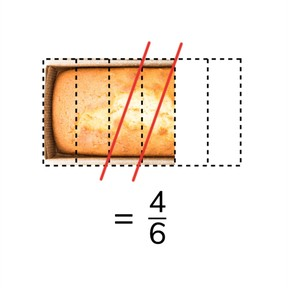Subtracting fractions with a common denominator

# Subtracting fractions with a common denominator

I can subtract simple fractions with common denominators.

No account needed.8,000 schools use Gynzy92,000 teachers use Gynzy1,600,000 students use Gynzy

## General

Students learn to subtract simple fractions with common denominators.

## Common core standard(s)

CCSS.Math.Content.4.NF.B.3a

## Relevance

Discuss with students that it is useful to be able to subtract fractions with common denominators so that you can determine how much you have left expressed as a fraction.

## Introduction

Show students a few images on the interactive whiteboard and ask students to determine the fractions that represent the given images. Ask students to explain their thinking.

## Development

Tell students that fractions with common denominators have denominators which are the same. Explain how to subtract fractions with common denominators using the cream pie on the interactive whiteboard. The cream pie is cut into 4 equal pieces. There are three pieces left. 1//4 is eaten. To know how much you have left you subtract the fractions. 3//4 - 1//4. Tell students that when subtracting, you subtract the numerators, and leave the denominators the same. The answer is 2//4 (which can be simplified to 1//2). Have students practice a few problems with visual support. Next, you can explain that subtraction without visual support is exactly the same, you just subtract the numerators. Have students practice with a few problems. You can also have mixed fractions. You first subtract the whole numbers, and then subtract the fractions. Have students practice with a few examples. Finally discuss how to solve fraction story problems. They must first find the fractions in the story and then solve by subtracting numerators. Do the first problem as a class and then have students practice the fraction word problems.

To check that students understand subtracting fractions with common denominators, ask students the following questions:
- When does a fraction have common denominators?
- What do you do when you subtract fractions with common denominators?

## Guided practice

Students are first given fraction subtraction problems with visual support, then they are asked to match fraction equations and their solutions, and finally are given fraction word problems to solve.

## Closing

Discuss that it is important to be able to subtract fractions with common denominators so you can calculate how many units you have left. Ask students to form pairs and to play memory. They must first create the memory cards by writing a subtraction equation on the first card and the difference on the second card. Then play memory.

## Teaching tips

Students who have difficulty with subtracting fractions with common denominators can first practice getting comfortable with the terms numerator and denominator. You can remind students to think of it as a "down"-ominator (so it belongs on the bottom). Then have students match fractions with common denominators, followed by subtracting them. When they are comfortable with simple fractions, have them work with mixed fractions

### The online teaching platform for interactive whiteboards and displays in schools

• Save time building lessons

• Manage the classroom more efficiently

• Increase student engagement